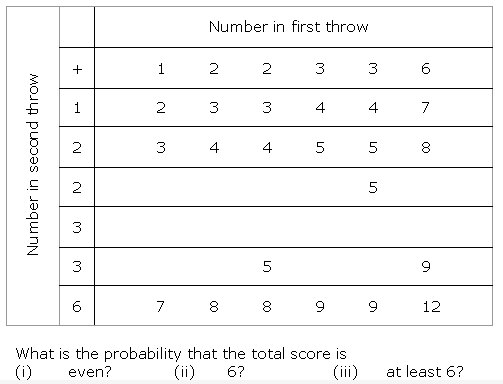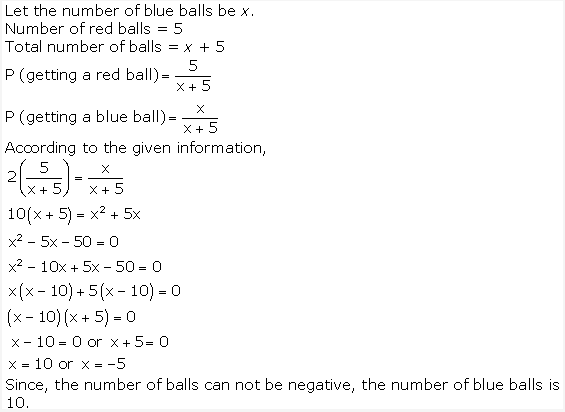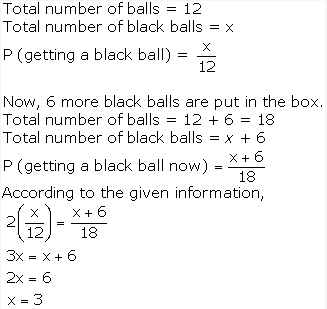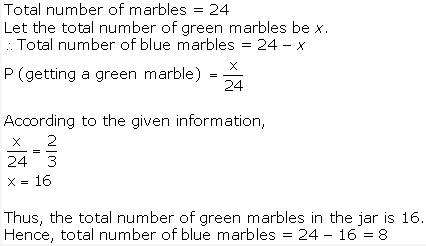NCERT Solutions for Class 10 Maths Chapter 15 Probability Ex 15.2 are part of NCERT Solutions for Class 10 Maths. Here we have given NCERT Solutions for Class 10 Maths Chapter 15 Probability Ex 15.2.

 Board CBSE Textbook NCERT Class Class 10 Subject Maths Chapter Chapter 15 Chapter Name Probability Exercise Ex 15.2 Number of Questions Solved 5 Category NCERT Solutions

## NCERT Solutions for Class 10 Maths Chapter 15 Probability Ex 15.2

Question 1.
Two customers Shyam and Ekta are visiting a particular shop in the same week (Tuesday to Saturday). Each is equally likely to visit the shop on any day as on another day. What is the probability that both will visit the shop on
(i) the same day?
(ii) consecutive days?
(iii) different days?
Solution:Question 2.
A die is numbered in such a way that its faces show the number 1, 2, 2, 3, 3, 6. It is thrown two times and the total score in two throws is noted. Complete the following table which gives a few values of the total score on the two throws:What is the probability that the total score is at least 6?
(i) even
(ii) 6
(iii) at least 6
Solution:Question 3.
A bag contains 5 red balls and some blue balls. If the probability of drawing a blue ball is doubles that of a red ball, determine the number of blue balls in the bag.
Solution:Question 4.
A box contains 12 balls out of which x are black. If one ball is drawn at random from the box, what is the probability that it will be a black ball? If 6 more black balls are put in the box, the probability of drawing a black ball is now double of what it was before. Find x.
Solution:Question 5.
A jar contains 24 marbles, some are green and others are blue. If a marble is drawn at random from the jar, the probability that it is green is 2/3. Find the number of blue balls in the jar.
Solution:We hope the NCERT Solutions for Class 10 Maths Chapter 15 Probability Ex 15.2, help you. If you have any query regarding NCERT Solutions for Class 10 Maths Chapter 15 Probability Ex 15.2, drop a comment below and we will get back to you at the earliest.